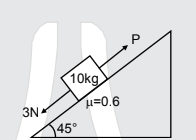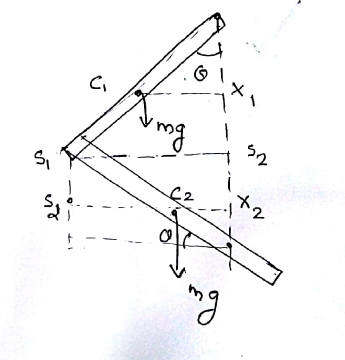#### A block of mass 10 kg is kept on a rough inclined as shown in figure. The coefficient of friction between the block and the surface is 0.6. Two forces of magnitude 3N & P Newton are acting of the block as shown figure. If friction on the block is acting upwards then minimum value of P for which the block remains at rest isUse3 + mg sin= P +mg cosP = 32 N

#### Safeer PP